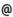## "Babes-Bolyai" University of Cluj-Napoca Faculty of Mathematics and Computer Science

 Numerical solutions of equations
 Code Semes-ter Hours: C+S+L Credits Type Section MO010 8 2+2+0 7.5 optional Matematică MO010 8 2+2+0 7.5 optional Matematică-Informatică
 Teaching Staff in Charge
 Assoc.Prof. DIACONU Adrian, Ph.D., adiaconumath.ubbcluj.ro
 Aims The presentation of certain notions and results concerning the principal iterativ methods and those convergence.
 Content Functional analysis elements in linear normed spaces. The linear normed space of the n-linear and continuous mappings between two linear normed spaces. The inversion of the linear and continuous mappings and the approximation of the inverted mappings ( The method of Schultz ). Applications of the prolongation theorems of the linear funtionals to the construction of a type of abstract interpolation polynomials Lagrange type, of the invertid interpolation polynomials and some approximation of the equations'solution. Differential calculus elements in linear normed spaces. The Frechet differential of a fonction defined between linear normed spaces. Taylor's abstract polynomial and Taylor type formula in the case of non-linear mappings defined between linear normed spaces. The approximation of the solutions of an operational equation with the aid of the abstract Taylor polynomial applied on the inverted function. The Chebischev Type iterative methods and their convergence. The Newton-Kantorovich method. The inclusion among the iterative Chebischev type methods. Kantorovich's theorem of convergence and of the existence of the solution. Cases in which the solution is unique. Applications for equations in the set of real numbers, for the determination of the values of implicite functions, for the approximation of the solutions of equation systems, diferential and integral equations. The approximation of the equations'solutions in Banach spaces with the aid approximant sequences. The notion of approximant sequence and that of its order. The convergence of the iterative Chebischev and Traub methods. Iterative methods which use devided abstract differences. Iterative methods which allow the simultaneous approximation of the solution of an operational equation and the inverse of a linear operator which intervenes.
 References 1. ARGYROS, I.,K., Polinomial Operator Equation in Abstract Spaces and Applications.-CRC Press Boca Raton, Boston, London, New-York, Washington D.C., 1998. 2. DIACONU, A., The acceleration of the convergence of certain approximant sequnces, PU.M.A. 11(2000), nr.2, 187-207. 3. KANTOROVICI, L.,V., AKILOV, G.,P., Analiza Functionala, Editura Stiintifica si Enciclopedica, Bucuresti, 1986. 4. PAVALOIU, I., Introducere in teoria aproximarii solutiilor ecuatiilor, Editura Dacia, Cluj-Napoca, 1976. 5. PAVALOIU,I., Rezolvarea ecuatiilor prin interpolare, Editura Dacia, Cluj-Napoca, 1981.
 Assessment Exam.